# GMAT Math : Equations

## Example Questions

← Previous 1

### Example Question #1 : Dsq: Solving Equations

If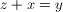, what is the value of?

(1)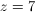(2)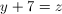Statement (1) ALONE is sufficient, but statement (2) alone is not sufficient.

BOTH statements TOGETHER are sufficient, but NEITHER statement ALONE is sufficient.

Statement (2) ALONE is sufficient, but statement (1) alone is not sufficient.

EACH statement ALONE is sufficient.

Statements (1) and (2) TOGETHER are NOT sufficient.

Statement (2) ALONE is sufficient, but statement (1) alone is not sufficient.

Explanation:Therefore,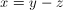(1) If, then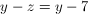, and the value of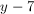can vary.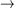NOT sufficient

(2) Subtracting bothand 7 from each side ofgives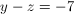.

The value of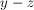can be determined.SUFFICIENT

### Example Question #131 : Algebra

If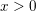, what is the value of x?

Statement 1: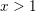Statement 2: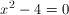EACH statement ALONE is sufficient.

Statements 1 and 2 TOGETHER are NOT sufficient.

Statement 1 ALONE is sufficient, but Statement 2 is not sufficient.

Statement 2 ALONE is sufficient, but Statement 1 is not sufficient.

BOTH statements TOGETHER are sufficient, but NEITHER statement ALONE is sufficient.

Statement 2 ALONE is sufficient, but Statement 1 is not sufficient.

Explanation:

We are looking for one value of x since the quesiton specifies we only want a positive solution.

Statement 1 isn't sufficient because there are an infinite number of integers greater than 1.

Statement 2 tells us that x = 2 or x = –2, and we know that we only want the positive answer. Then Statement 2 is sufficient.

### Example Question #931 : Data Sufficiency Questions

What is the value of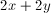?

Statement 1: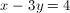Statement 2: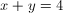EACH statement ALONE is sufficient.

Statement 2 ALONE is sufficient, but statement 1 is not sufficient.

Statement 1 ALONE is sufficient, but statement 2 is not sufficient.

BOTH statements TOGETHER are sufficient, but NEITHER statement ALONE is sufficient.

Statements 1 and 2 TOGETHER are NOT sufficient.

Statement 2 ALONE is sufficient, but statement 1 is not sufficient.

Explanation:

We know that we need 2 equations to solve for 2 variables, so it is tempting to say that both statements are needed. This is actually wrong! We aren't being asked for the individual values of x and y, instead we are being asked for the value of an expression.is just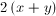, and statement 2 gives us the value of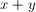. For data sufficiency questions, we don't actually have to solve the question, but if we wanted to, we would simply multiply statement 2 by 2.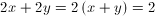* Statement 2 =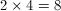### Example Question #4 : Equations

Data Sufficiency Question- do not actually solve the problem

Solve for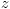.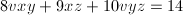1.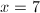2.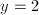Both statements taken together are suffienct to answer the question, but neither statement alone is sufficient

Statement 2 alone is sufficient, but statement 1 alone is not sufficient to answer the question

Statement 1 alone is sufficient, but statement 2 alone is not sufficient to answer the question

Each statement alone is sufficient

Statements 1 and 2 together are not sufficient, and additional data is needed to answer the question

Statements 1 and 2 together are not sufficient, and additional data is needed to answer the question

Explanation:

In order to solve an equation with 4 variables, you need to know either 3 of the variables or have a system of 4 equations to solve.

### Example Question #1 : Equations

Solve for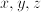.

Statement 1: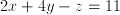Statement 2: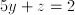Statements 1 and 2 TOGETHER are NOT sufficient.

EACH statement ALONE is sufficient.

Statement 1 ALONE is sufficient, but statement 2 is not sufficient.

Statement 2 ALONE is sufficient, but statement 1 is not sufficient.

BOTH statements TOGETHER are sufficient, but NEITHER statement ALONE is sufficient.

Statements 1 and 2 TOGETHER are NOT sufficient.

Explanation:

To solve for three unknowns, we need three equations.  Therefore no combination of statements 1 and 2 will provide enough information to solve for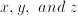.

### Example Question #1 : Equations

Solve for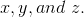Statement 1: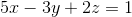Statement 2: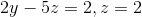Statements 1 and 2 TOGETHER are NOT sufficient.

EACH statement ALONE is sufficient.

BOTH statements TOGETHER are sufficient, but NEITHER statement ALONE is sufficient.

Statement 1 ALONE is sufficient, but statement 2 is not sufficient.

Statement 2 ALONE is sufficient, but statement 1 is not sufficient.

BOTH statements TOGETHER are sufficient, but NEITHER statement ALONE is sufficient.

Explanation:

To solve for three unknowns, we need three equations.  Here we have three equations if we use both statements 1 and 2.  We don't need to solve any further.  Because this is a data sufficiency question, it doesn't matter what the actual values of x, y, and z are.  The important fact is the we could find them if we wanted to.

### Example Question #1 : Dsq: Solving Equations

The volume of a fixed mass of gas varies inversely with the atmospheric pressure, in millibars, acting upon it, given that all other conditions remain constant.

At 12:00, a balloon was filled with exactly 100 cubic yards of helium. What is its current volume?

Statement 1: It is now 2:00.

Statement 2: The atmospheric pressure is now 105 millibars.

EITHER statement ALONE is sufficient to answer the question.

BOTH statements TOGETHER are sufficient to answer the question, but NEITHER statement ALONE is sufficient to answer the question.

BOTH statements TOGETHER are insufficient to answer the question.

Statement 1 ALONE is sufficient to answer the question, but Statement 2 ALONE is NOT sufficient to answer the question.

Statement 2 ALONE is sufficient to answer the question, but Statement 1 ALONE is NOT sufficient to answer the question.

BOTH statements TOGETHER are insufficient to answer the question.

Explanation:

The first statement is unhelpful; the time of day is irrelevant to the question.

You can use the following variation equation to deduce the current volume:or, equivalently,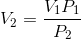To find the current volume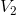, you therefore need three things - the initial volume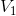, which is given in the body of the question; the current pressure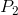, which you know if you use Statement 2, and the initial pressure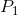, which is not given anywhere.

### Example Question #1 : Dsq: Solving Equations

The electrical current through an object in amperes varies inversely as the object's resistance in ohms, given that all other conditions are equal.

Four batteries hooked up together run an electrical current of 3.2 amperes through John's flashlight. How much current would the same batteries run through John's radio?

Statement 1: The radio has resistance 20 ohms.

Statement 2: The flashlight has resistance 15 ohms.

BOTH statements TOGETHER are insufficient to answer the question.

Statement 2 ALONE is sufficient to answer the question, but Statement 1 ALONE is NOT sufficient to answer the question.

Statement 1 ALONE is sufficient to answer the question, but Statement 2 ALONE is NOT sufficient to answer the question.

EITHER statement ALONE is sufficient to answer the question.

BOTH statements TOGETHER are sufficient to answer the question, but NEITHER statement ALONE is sufficient to answer the question.

BOTH statements TOGETHER are sufficient to answer the question, but NEITHER statement ALONE is sufficient to answer the question.

Explanation:

Let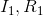be the current through and the resistance of the flashlight; let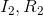be the current through and the resistance of the radio.

The variation equation here would be:or equivalently: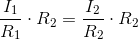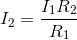So in order to find the current in the radio, you need to know three things - the current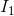in the flashlight, which you know from the body of the problem; the resistance from the flashlight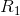, which you know if you are given Statement 2; and the resistance from the radio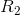, which you know if you are given Statement 1. Just substitute and solve.

### Example Question #1 : Dsq: Solving Equations

Evaluate: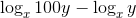Statement 1: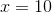Statement 2: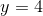BOTH statements TOGETHER are sufficient to answer the question, but NEITHER statement ALONE is sufficient to answer the question.

BOTH statements TOGETHER are insufficient to answer the question.

Statement 1 ALONE is sufficient to answer the question, but Statement 2 ALONE is NOT sufficient to answer the question.

Statement 2 ALONE is sufficient to answer the question, but Statement 1 ALONE is NOT sufficient to answer the question.

EITHER statement ALONE is sufficient to answer the question.

Statement 1 ALONE is sufficient to answer the question, but Statement 2 ALONE is NOT sufficient to answer the question.

Explanation:

The difference of two logarithms with the same base is the same-base logarithm of the quotient of the numbers. Therefore, we can simplify this expression as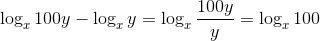We need only know the value of, given to us in Statement 1, in order to evaluate this expression.

### Example Question #2 : Dsq: Solving Equationsis a positive integer

True or false?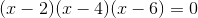Statement 1: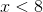Statement 2:is even.

Statement 1 ALONE is sufficient to answer the question, but Statement 2 ALONE is NOT sufficient to answer the question.

EITHER statement ALONE is sufficient to answer the question.

Statement 2 ALONE is sufficient to answer the question, but Statement 1 ALONE is NOT sufficient to answer the question.

BOTH statements TOGETHER are insufficient to answer the question.

BOTH statements TOGETHER are sufficient to answer the question, but NEITHER statement ALONE is sufficient to answer the question.

BOTH statements TOGETHER are sufficient to answer the question, but NEITHER statement ALONE is sufficient to answer the question.

Explanation:

By the zero product principle, we can solve by setting each linear binomial to zero and solving. This yields three solutions: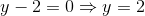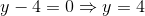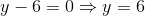Neither statement alone narrowsto one of these three solutions, but the two together do.

← Previous 1

### All GMAT Math Resources